时间：2020-02-10  来源：百度经验  作者：Vouzou  阅读：7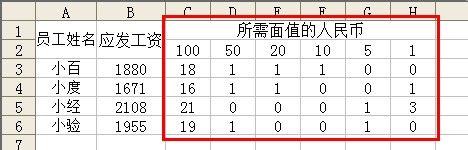“MOD(B3,\$C\$2)”表示取B3单元格数值被C2单元格的数值取整所剩的余数；“=INT(MOD(B3,\$C\$2)/\$D\$2)”表示取“MOD(B3,\$C\$2)”与D2单元格的数值相除，并对其结果取整数。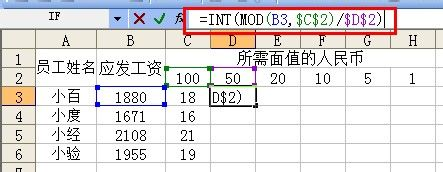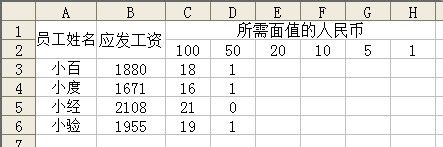“MOD(MOD(B3,\$C\$2),\$D\$2)”表示取“MOD(B3,\$C\$2)”被D2单元格的数值取整所剩的余数；“=INT(MOD(MOD(B3,\$C\$2),\$D\$2)/\$E\$2)”表示取“MOD(MOD(B3,\$C\$2),\$D\$2)”与E2单元格的数值相除，并对其结果取整数。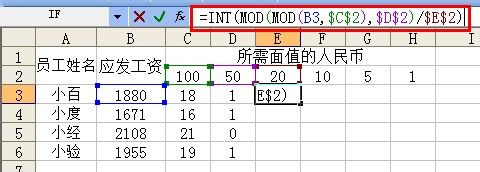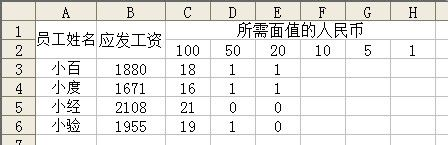F3中输入“=INT(MOD(MOD(MOD(B3,\$C\$2),\$D\$2),\$E\$2)/\$F\$2)”G3中输入“=INT(MOD(MOD(MOD(MOD(B3,\$C\$2),\$D\$2),\$E\$2),\$F\$2)/\$G\$2)”

H3中输入“=INT(MOD(MOD(MOD(MOD(MOD(B3,\$C\$2),\$D\$2),\$E\$2),\$F\$2),\$G\$2)/\$H\$2)”